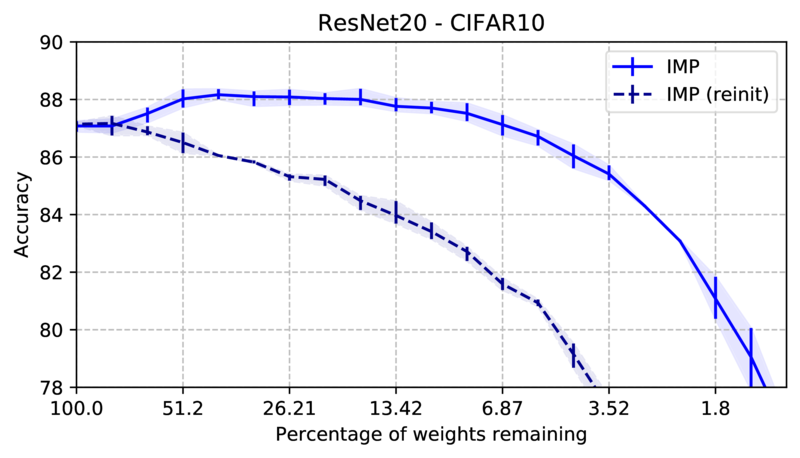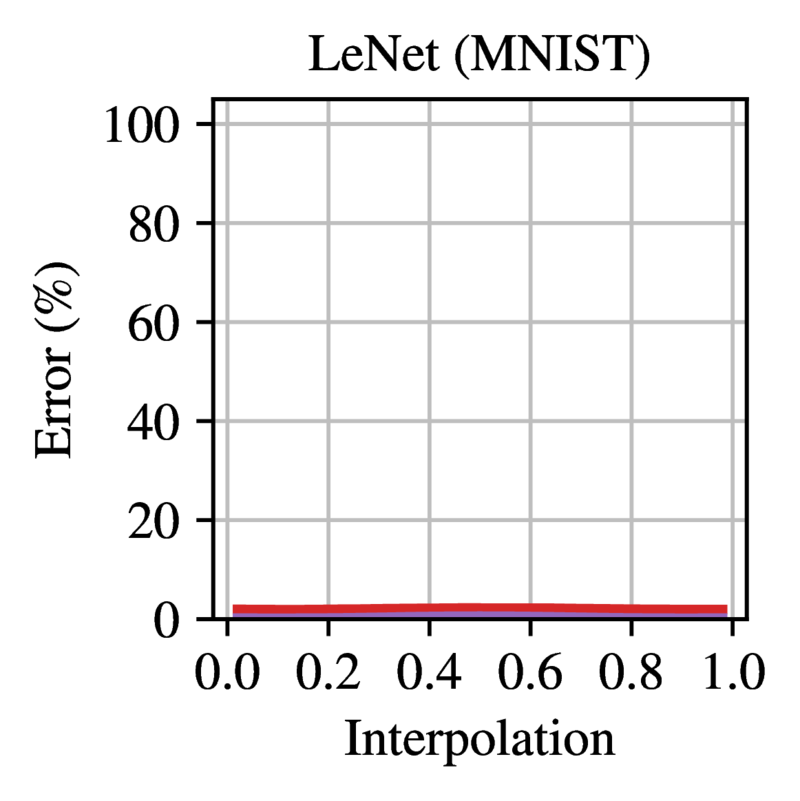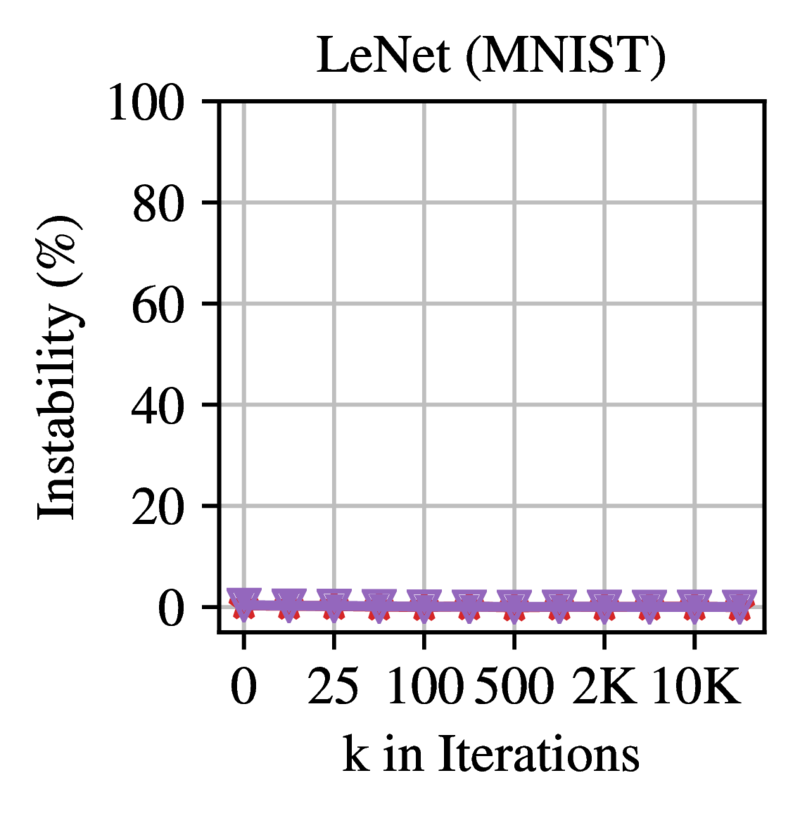# On the Lottery ticket hypothesis

In this post I aim to give a condensed overview of the current research on the lottery ticket hypothesis (LTH). As most of this is quite recent work, I’ve found there are few comprehensive resources as of now, and knowledge is mostly still scattered around in papers. For other sources, I can recommend:

• The original papers on the LTH by Frankle et al. (1, 2). They are excellently written and contain very in-depth appendices.
• A survey by R. Lange, summarizing a lot of the core papers on the LTH.

I’ll assume basic familiarity with neural network pruning. This post has been based on a chapter from my master thesis.

## The lottery ticket hypothesis

It was commonly believed that sparse training from scratch is infeasible, until a landmark paper by Frankle and Carbin (2018) introduced the lottery ticket hypothesis. Through extensive experimentation, the authors show that a sparse network can train to the same accuracy as a dense network, as long as a specific initialization is used. Based on this observation, Frankle and Carbin (2018) formulate the following hypothesis:

The Lottery Ticket Hypothesis. A randomly-initialized, dense neural network contains a subnetwork that is initialized such that — when trained in isolation — it can match the test accuracy of the original network after training for at most the same number of iterations.

This implies that, if only the correct subnet can be determined, we can train the sparse subnet instead of the dense network. A hopeful result, although the method to find the subnet in question is very expensive. It consists of an iterative pruning algorithm, where non-pruned weights are reset back to their initialization before retraining. This is called iterative magnitude pruning (IMP).

Algorithm 1: Iterative magnitude pruning
Initialize the network $\mathcal{N}$ with random weights $\boldsymbol{\theta}_0$. Create the mask $\boldsymbol{m}$ := $1^d$.
while desired sparsity not achieved do
$\qquad$Train $\boldsymbol{\theta}_0 \odot \boldsymbol{m}$ for $i$ iterations, arriving at weights $\boldsymbol{\theta}_i \odot \boldsymbol{m}$.
$\qquad$Prune $p\%$ of the connections not masked by $\boldsymbol{m}$ with the smallest magnitude in $\boldsymbol{\theta}_i$. Set these connections to $0$ in the mask $\boldsymbol{m}$.

Figure 1 gives a basic demonstration of IMP. At each pruning round, the trained weights are used to determine the new sparser mask, while the remaining weights always start from the same initialization. As a comparison, using the exact same structure with a new initialization (usually called a reinitialization, or reinit) reaches significantly lower performance. This is the novel part of IMP compared to prior sparse training attempts; the weights need to be the same as used when pruning.Figure 1: Basic example of the lottery ticket hypothesis on a residual network. A first experiment performs iterative magnitude pruning. Each round the network is trained, after which the 20% smallest weights are removed and the remaining weights are reset to the initialization. In a second experiment we train the same network structures, but with a new reinitialization instead of the original initialization. Experiments on CIFAR-10 with ResNet20. Average of 3 runs with different seeds; error bars indicate maximum and minimum values.

The lottery ticket hypothesis also holds to some extent using one-shot pruning, but Frankle and Carbin (2018) show that the strongest results are achieved when iteratively pruning and retraining with an exponentially decaying sparsity schedule. In practice, pruning $20\%$ per round is the usual compromise between accuracy and speed. This means that IMP is an exceedingly slow algorithm to prune a network; achieving $99\%$ sparsity requires fully training the model 20 times (as $0.8^{20} \approx 0.01$). This is further exacerbated by the fact that IMP is not parallelizable.

The LTH sparked many follow-up questions. Why is the initialization so important combined with the structure. What are the properties of these lottery tickets? Does it hold in all cases? Are lottery tickets specific to a certain dataset or optimizer?

In the original paper, Frankle and Carbin (2018) conjecture (but do not prove) that SGD seeks out a small subnet (the lottery ticket) during training and optimizes this. IMP finds these small subnets and cuts away all the superfluous parts of the model, which explains why very sparse networks can still rival the dense performance. According to this interpretation, over-parameterized networks are easier to train because they contain more subnets, and hence have more possible winning tickets at initialization.

## Linear mode connectivity

To find lottery tickets in medium-sized networks Frankle and Carbin (2018) need to use warm-up and a small learning rate. Liu et al. (2018) and Gale et al. (2019) show that lottery tickets could not be found in large-scale networks such as ResNet50 on ImageNet, further calling the generality of the LTH into question. This prompted Frankle et al. (2020) to devise a refinement to the lottery ticket hypothesis, relying upon the concept of mode connectivity.

Mode connectivity is introduced to study the (in)stability of stochastic training. Neural network optimization introduces noise into training through the random ordering of batches and possibly also via data augmentations (e.g. random flips of images). By training the network twice from the same starting point with different noise, one can determine if the resulting optima are connected as a proxy for stability.

Two networks are called mode connected if there exists a path between them in weight space that does not increase the error. Linear mode connectivity requires the path between the modes to be linear.

More formally let $\mathcal{E}(\boldsymbol{\theta})$ be the error of the network with weights $\boldsymbol{\theta}$. Frankle et al. (2020) define the linearly interpolated error between $\boldsymbol{\theta}_1$ and $\boldsymbol{\theta}_2$ as $\mathcal{E}_\alpha (\boldsymbol{\theta}_1, \boldsymbol{\theta}_2) = \mathcal{E}(\alpha \cdot \boldsymbol{\theta}_1 + (1-\alpha) \cdot \boldsymbol{\theta}_2)$, with $\alpha \in [0, 1]$. The linear interpolation instability (LII) is the highest loss increase on this path:

$LII(\boldsymbol{\theta}_1, \boldsymbol{\theta}_2) = \sup_\alpha \, \mathcal{E}_\alpha (\boldsymbol{\theta}_1, \boldsymbol{\theta}_2) - \frac {\mathcal{E}(\boldsymbol{\theta}_1) + \mathcal{E}(\boldsymbol{\theta}_2)} 2$

The LII is used to check the stability of networks towards SGD noise. When training a network twice using a different data ordering, a network is said to be stable to SGD noise when these two networks after training have a LII close to zero. In practice, the LII will rarely be exactly zero, so a small threshold such as 2% can be used as a measure for empirical stability.

Frankle et al. (2020) show that very small networks such as LeNet are already stable at initialization, while larger networks become stable in an early phase during training. Figure 2 shows the error on the linear path between two dense networks trained from the same initialization with different SGD noise. All models except LeNet are completely unstable at initialization, achieving random error between the two modes. Figure 3 shows how the stability evolves of dense models during training. The LII becomes close to zero after a short period in early training.Figure 2: Error on the linear path between networks trained from the same initialization with different SGD noise. Trained networks are at 0.0 and 1.0. Each line is the mean and standard deviation across three initializations and three data orders (nine samples in total). Reprinted from Frankle et al. (2020).Figure 3: Linear interpolation instability when starting from step k. Each line is the mean and standard deviation across three initializations and three data orders (nine samples in total). Reprinted from Frankle et al. (2020).

Frankle et al. (2020) connect this discussion on stability with the LTH, by showing through extensive experiments that the hypothesis only applies when resetting to a stable state. So instead of resetting entirely to initialization each round, they rewind to an early epoch where the training has become stable. This revised version of the LTH is also called the stabilized lottery ticket hypothesis.

Although they originally focussed on computer vision tasks, subsequent works have since shown that the stabilized LTH holds on a very wide range of models and tasks, such as BERT (Chen et al, 2020), self-supervised computer vision tasks (Chen et al, 2021), adversarial training (Li et al, 2020), LSTM’s and reinforcement learning (Yu et al, 2020).

## Pruning as training and the supermask

The reliance of the lottery tickets on the original initialization leads to questions on the nature of these initializations. Frankle and Carbin (2018) hypothesize that lottery tickets encode useful inductive biases for the problem and the optimizer. However, Zhou et al. (2019) find that lottery tickets can already exhibit non-trivial accuracy without training, indicating that they contain a stronger prior than what Frankle and Carbin (2018) implied.

Zhou et al. (2019) continue by designing a training method that does not update weights, but only removes them. Given the huge number of parameters in a dense network, and that a network has in the order of $2^{|\boldsymbol{\theta}|}$ subnetworks, the authors propose that there exists a performant subnet hidden in every randomly-initialized network. Ramanujan et al. (2020) improve on this algorithm and show that even for more challenging tasks such as ImageNet, they can find subnets with fairly competitive accuracies. These performant subnets of random networks are called supermasks. Based on this empirical evidence, Ramanujan et al. (2020) state a stronger version of the LTH:

The Strong Lottery Ticket Hypothesis. A randomly-initialized, dense neural network $\mathcal{N}_1$ contains a subnetwork $\mathcal{N}_2$ that is initialized such that — without training — it can match the test accuracy of $\mathcal{N}_1$ after training.

The supermask methods show that pruning can be regarded as a method of training. Pruning simply constrains the hypothesis space to the axis-parallel projections of the full network, which can still be vast as long as the network is sufficiently over-parameterized. The work of Malach et al. (2020) gives a theoretical foundation to this observation, by proving a restricted version of the strong LTH. They prove that any dense ReLU network of depth $l$, can be approximated with an arbitrarly high probability, by a subnet of a random $2l$-layer network with sufficient width. Contrary to the proposition of the strong LTH in Ramanujan et al. (2020), the proof requires polynomial over-parametrization of the random network width, compared to the target network, limiting the practical implications. Orseau et al. (2020) improve this to a logarithmic bound, and removed the double depth requirement.

Though pruning random initialized networks is not necessarily useful in practice, it reshapes our understanding of pruning. Pruning is not only a compression method but also expressive enough to be a training method. This indicates that IMP might encode much bigger priors in lottery tickets then the IMP algorithm would reveal at first glance. This raises the concern that a lottery ticket might already contain all information of the solution. In the next section, we discuss the transferability of lottery tickets, to investigate how specifically these are tailored to the task or optimization process.

## Transferability of lottery tickets

Finding lottery tickets using IMP can be exceedingly expensive. Hence a natural question is whether a ticket transfers to other environments. Can a lottery ticket found using a certain optimizer also train to commensurate accuracy using another optimizer? And do they transfer across datasets, or even tasks?

Morcos et al. (2019) investigate precisely these questions on common vision tasks. They find that lottery tickets transfer fairly well across optimizers and datasets. Furthermore, winning tickets found on larger datasets (e.g. ImageNet or Places365) perform consistently better on small vision tasks (e.g. CIFAR10 or FashionMNIST), compared to the tickets generated on these tasks themselves. Sabatelli et al. (2020) also evaluate transferability on more diverse vision tasks with comparable results.

Chen et al. (2020) consider lottery ticket transferability in NLP settings. There is already an established practice in NLP to pretrain large language models such as BERT. The large upfront cost is amortized by transfer learning to a variety of downstream tasks. Chen et al. (2020) propose to use IMP in a similar fashion: the costly procedure of finding a ticket is performed once on a masked language modeling task, after which the ticket can be trained on a downstream task. They empirically show that BERT has universal lottery tickets at 70% sparsity, meaning they can transfer to tasks from GLUE and SQUAD while maintaining accuracy. In contrast, tickets found on downstream tasks did not transfer to most other tasks.

These results indicate that tickets found by IMP are reasonably general; structural transfer learning gives similar results compared to the established weight-based form of transfer learning.